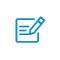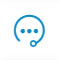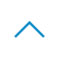# 四大机器学习编程语言对比：R、Python、MATLAB、Octave

GitHub 地址：https://github.com/mjbahmani/10-steps-to-become-a-data-scientist

R语言

R 是一种用于统计计算和图的语言及环境。它是一个 GNU 项目，与贝尔实验室的 John Chambers 及其同事开发的 S 语言及环境类似。R 可以视为 S 的一种不同实现。二者存在一些重要差异，但使用 S 写的很多代码在 R 下运行时无需修改。

• 端到端开发到执行(一些 brokers package 允许执行，IB)
• 开发速度快(比 Python 的代码少 60%)
• 开源包多
• 成熟的量化交易包(quantstrat、quantmod、performanceanalyitics、xts)
• 社区最大
• 使用 rcpp 可以整合 R 和 C++/C

• 比 Python 慢，尤其是在迭代循环和非向量化函数中
• 比 Matlab 绘图差，难以实现交互式图表
• 创建独立应用程序的能力有限

Python

Python 是一种用于通用编程的解释型高级编程语言，由 Guido van Rossum 创建并于 1991 年首次发布。Python 的设计强调代码可读性，使用了大量空格。它的结构使其在大规模和小规模编程中都能清晰明了。

• 端到端开发到执行(一些 brokers package 允许执行，IB)
• 开源包(Pandas、Numpy、scipy)
• 最适合一般编程和应用程序开发
• 可连接 R、C++ 和其他语言的「胶水」语言
• 总体速度最快，尤其是在迭代循环中

• 有一些不成熟的包，尤其是交易包
• 有些包与其他包不兼容或包含重叠
• 在金融领域的社区比 R 小
• 与 R 或 Matlab 相比，相同操作需要更多代码
• 追踪静默错误(silent error)可能需要很长时间(即使使用可视化调试器/IDE)

MATLAB

MATLAB(matrix laboratory)是一种多范型数值计算环境。作为 MathWorks 开发的一种专用编程语言，MATLAB 允许矩阵运算、函数和数据绘图、算法实现、用户界面创建，以及与用其他语言(包括 C、C++、C#、Java、Fortran、Python)写成的程序进行交互。

• 最快的数学和计算平台，尤其是向量化运算/线性矩阵代数。
• 适合所有数学和交易领域的商业级软件。
• 脚本简短，但高度集成了所有包。
• 拥有图和交互式图表的最佳可视化
• 具备良好测试和支持。
• 易于管理多线程支持和垃圾收集
• 最好的调试器

• 无法执行，必须转换成另一种语言。
• 昂贵：每个 license 大约 1000 美元，每添加一个包需要额外支付 50+ 美元。
• 无法与其他语言很好地集成。
• 很难检测出交易系统中的偏差(它是为数学和工程模拟而构建的)，因此可能需要广泛的测试。
• 糟糕的迭代循环性能。
• 无法开发单独的应用。

Octave

Octave 可以看作是商业语言 MATLAB 的 GNU 版本，它是一种脚本矩阵语言(scripting matrix language)，其语法有大约 95% 可与 MATLAB 兼容。Octave 由工程师设计，因此预装了工程师常用的程序，其中很多时间序列分析程序、统计程序、文件命令和绘图命令与 MATLAB 语言相同。

• 首先，目前没有可用的鲁棒性 Octave 编译器，且没有必要有，因为该软件可以免费安装。
• Octave 和 Matlab 的语言元素相同，除了一些个例，如嵌套函数。Octave 仍然处于积极开发的状态，每一个偏离 Matlab 语法之处都被视为 bug 或者至少是待解决问题。
• Octave 有很多可用工具箱，只要程序不要求图输出，那么在不进行大量更改的前提下，使用 Octave 运行和使用 Matlab 运行差不多。
• 图方面的能力是 Matlab 的优势。Matlab 最新版本包括 GUI 设计器，包含大量很棒的可视化特征。
• Octave 使用 GNU Plot 或 JHandles 作为图程序包，JHandles 与 Matlab 中的图程序包更接近一些。但是，Octave 不具备类似 GUI 设计器的组件，其可视化机制很受限且不与 Matlab 兼容。
• 集成开发环境也是类似的情况：Octave 有一个 QTOctave 项目，但仍处于早期阶段。
• Octave 社区的合作很可能帮助该软件很快提供更好、更兼容的图以及 GUI 能力。

https://towardsdatascience.com/r-vs-python-vs-matlab-vs-octave-c28cd059aa69扫码入群咨询反馈返回顶部